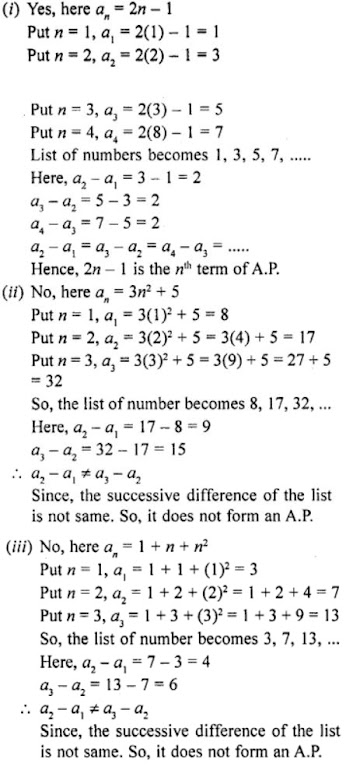# RD Sharma Solutions for Class 10 Maths Chapter 9 Arithmetic Progression Exercise 9.2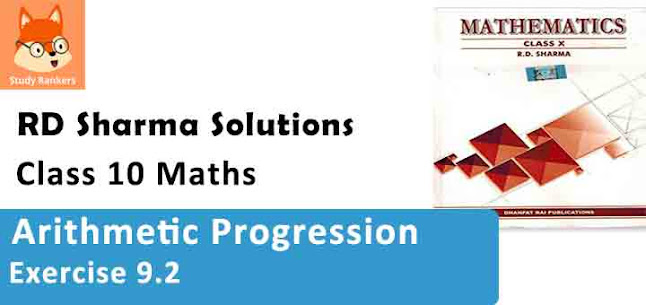1. For the following arithmetic progressions write the first term a and the common difference d:
(i) - 5, -1, 3,7, ........
(ii) 1/5, 3/5, 5/5, 7/5, .......
(iii) 0.3, 0.55, 0.80, 1.05, ......
(iv) -1.1, -3.1, -5.1, -7.1, .....

Solution

a is the first term and d is the common difference.
Arithmetic progression is a, a +d, a + 2d + a + 3d, .....

(i) -5, -1, 3,7, .....
Given arithmetic series is
-5, -1, 3,7, .....
This is in the form of a, a + d, a + 2d + a + 3d, .... by comparing these two
a = -5, a + d = 1, a + 2d = 3, a + 3d= 7, ....
First term (a) = -5
By subtracting second and first term, we get
(a + d) - (a) = d
⇒ -1-(-5) = d
⇒ 4 = d
Common difference (d) = 4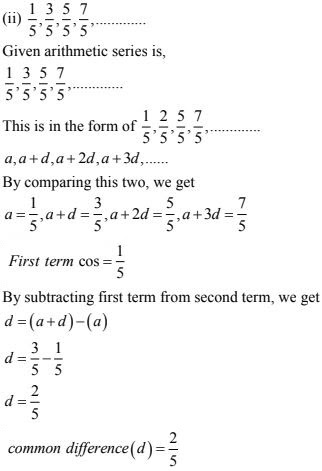(iii) 0.3, 0.55, 0.80, 1.05, .......
Given arithmetic series,
0.3, 0.55, 0.80,1.05, .....
General arithmetic series
a, a +d, a + 2d, a + 3d,....
By comparing,
a = 0.3, a + d = 0.5, a + 2d = 0.80, a + 3d = 1.05
First term(a) = 0.3
By subtracting first term from second term. We get
d = (a + d) - (a)
⇒ d = 0.55 - 0.3
⇒ d = 0.25
Common difference (d) = 0.25

(iv) -1.1, -3.1, -5.1, -7.1,....
General series is
a, a + d, a + 2d, a+ 3d, .....
By comparing this two, we get
a = -1.1, a + d= -3.1, a + 2d= -5.1, a + 3d = -71
First term (a) = -1.1
Common difference (d) = (a + d) - (a)
= -3.1 - (-1.1)
Common difference (d) = -2

2. Write the arithmetic progressions write first term a and common difference d are as follows:
(i) a = 4, d = -3
(ii) a = -1, d = 1/2
(iii) a = -1.5, d = -0.5

Solution

If first term (a) = a and common difference = d, then the arithmetic series is, a, a + d, a + 2d, a + 3d, .....

(i) a = 4, d = -3
Given first term (a) = 4
Common difference(d) = -3
Then arithmetic progression is,
a, a+ d, a + 2d, a+ 3d, .....
⇒ 4, 4 - 3, a + 2(-3), 4 + 3(-3).....
⇒ 4, 1, -2; -5, -8,....

(ii) Given , First term(a) = -1
Common difference(d) = 1/2
Then arithmetic progression is,
⇒ a, a+d, a+ 2d, a+ 3d, .....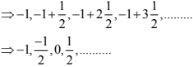(iii) Given, First term(a) = -1.5
Common difference(d) = -0.5
Then arithmetic progression is
⇒ a, a + d, a +2d, a + 3d,....
⇒ -1.5, -1.5 - 0.5, -1.5+2(-0.5), -1.5 + 3(-0.5)
⇒ -1.5, -2, -2.5, -3,....
Then required progression is
-1.5, -2, -2.5, -3,....

3. In which of the following situations, the sequence of numbers formed will form an A.P.?
(i) The cost of digging a well for the first metre is Rs 150 and rises by Rs 20 for each succeeding metre.
(ii) The amount of air present in the cylinder when a vacuum pump removes each time 1/4 of their remaining in the cylinder.

Solution

(i) Given,
Cost of digging a well for the first meter(c1) = Rs. 150.
Cost rises by Rs. 20 for each succeeding meter
Then,
Cost of digging for the second meter (c2)= Rs. 150 + Rs 20 = Rs 170
Cost of digging for the third meter (c3) = Rs. 170 + Rs 20 = Rs 190
Thus, costs of digging a well for different lengths are 150, 170, 190, 210,......
Clearly, this series in in A.P.
With first term (a) = 150, common difference(d) = 20

(ii) Given,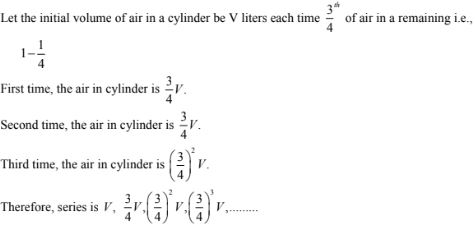4. Show that the sequence defined by an = 5n - 7 is an A.P., find its common difference.

Solution

Given sequence: an = 5n - 7
nth term of given sequence (an) = 5n - 7
(n+1)th term of given sequence (an + 1) - an
= (5n - 2) - (5n - 7)
= 5
∴ d = 5
OR

an = 5n - 7
Let n= 1, 2, 3, 4...., we get
a1 = 5 × 1 - 7 = 5 - 7 = -2
a2 = 5 × 2 - 7 = 10 - 7 = 3
a3 = 5 × 3 - 7 = 15 - 7 = 8
a4 = 5 × 4 - 7 = 20 - 7 = 13,.....
-2, 3, 8, 13,.... is an A.P. whose common difference is 5 as
a2 - a1 = 3-(-2) = 3 + 2 = 5
a3 - a2 = 8-3 = 5
a4 - a3 = 13 - 8 = 5

5. Show that the sequence defined by an = 3n2 - 5 is not an A.P.
Solution
Given sequence is:
an = 3n2 - 5
Let n = 1, 2, 3, 4,....we get
a1 = 3(1)2 - 5 = 3×1 - 5 = 3-5 = -2
a2 = 3(2)2 - 5 = 3×4 - 5 = 12-5 = 7
a3 = 3(3)2 - 5 = 3×9 - 5 = 27-5 = 22
a4 = 3(4)2 - 5 = 3×16 - 5 = 48-5 = 43
The sequence will be -2, 7, 22, 43,....
We see that it has no common difference.
It is not an A.P.

6. The general term of a sequence is given by an = -4n + 15. Is the sequence an A.P. ? If so, find its 15th term and the common difference.
Solution
Let n = 1, 2, 3, 4, 5,.... then
a1 = -4×1 + 15 = -4+15 = 11
a2 = -4×2 + 15 = -8+15 = 7
a3 = -4×3 + 15 = -12+15 = 3
a4 = -4×4 + 15 = -16+15 = -1
a5 = -4×5 + 15 = -20+15 = -5
We see that first term is 11 and common difference is -4
a2 - a1 = 7-11 = -4
a3 - a2 = 3-7 = -4
a4 - a3 = -1-3 = -4
a5 - a4 = -5-(-1) = -5+1 = -4
Yes, it is an A.P.
Now, 15th term = n15 = -4×15 + 15 = -60+15 = -45

7. Write the sequence with nth term :
(i) an = 3 + 4n
(ii) an = 5 + 2n
(iii) an = 6 – n
(iv) an = 9 – 5n
Show that all of the above sequences form A.P.
Solution

(i) nth term
an = 3 + 4n
Let n = 1, 2, 3, 4,.... we get
a1 = 3 + 4×1 = 3+4 = 7
a2 = 3 + 4×2 = 3+8 = 11
a3 = 3 + 4×3 = 3+12 = 15
a4 = 3 + 4×4 = 3+16 = 19
We see that the sequence is 7, 4, 15, 19,....
Here, common difference a2 - a1 = 11 - 7 = 4 is same for the sequences.
Therefore, this sequence is an A.P

(ii) nth term, an = 5 + 2n
Let n = 1, 2, 3, 4,.... we get
a1 = 5 + 2×1 = 5+2 = 7
a2 = 5 + 2×2 = 5+4 = 9
a3 = 5 + 2×3 = 5+6 = 11
a4 = 5 + 2×4 = 5+8 = 13
We see that the sequence is 7, 9, 11, 13,....
Here, common difference a2 - a1 = 9 - 7 = 2 is same for the sequences.
Therefore, this sequence is an A.P

(iii) nth term, an = 6 - n
Let n = 1, 2, 3, 4, 5,....we get,
a1 = 6 - 1 = 5
a2 = 6 - 2 = 4
a3 = 6 - 3 = 3
a4 = 6 - 4 = 2
a5 = 6 - 5 = 1
We see that the sequence is 5, 4, 3, 2, 1,....
Here, common difference a2 - a1 = 4 - 5 = -1 is same for the sequences.
Therefore, this sequence is an A.P.

(iv) nth term, an = 9 - 5n
Let n = 1, 2, 3, 4, 5,.... then
a1 = 9 - 5×1 = 9-5 = 4
a2 = 9 - 5×2 = 9-10 = -1
a3 = 9 - 5×3 = 9-15 = -6
a4 = 9 - 5×4 = 9-20 = -11
a5 = 9 - 5×5 = 9-25 = -16
We see that the sequence is 4, -1, -6, -11, -16,....
Here, common difference a2 - a1 = -1 - 4 = -5 is same for the sequences.
Therefore, this sequence is an A.P.

8. Find the common difference and write the next four terms of each of the following arithmetic progressions:

(i) 1, -2, -5, -8, .......
(ii) 0, -3, -6, -9, .......
(iii) -1, 1/4, 3/2,.......
(iv) -1, -5/6, -2/3,.......

Solution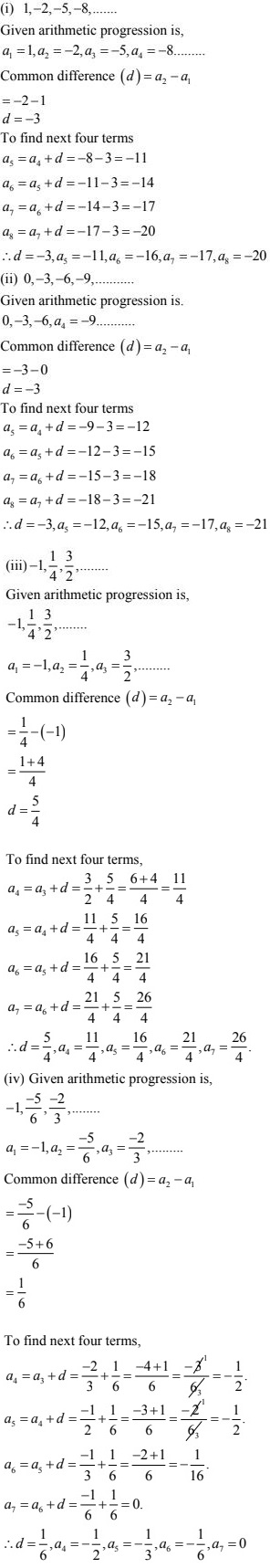8. Prove that no matter what the real numbers a and b are, the sequence with nth term a + nb is always an A.P. What is the common difference ?

Solution

Given sequence (an) = an + 6n
nth term (an) = a + nb
(n +1)th term (an+1) = a + (n+1)b.
Common difference (d) = an+1 - an
d = (a +(n +1)b)-(a + nb)
= a + nb + b - a - nb
= b
∴ common difference (d) does not depend on nth value so, given sequence I sin AP with (d) = b

10. Find out which of the following sequences are arithmetic progressions. For those which are arithmetic progressions, find out the common differences.
(i) 3, 6, 12, 24; ......
(ii) 0, -4, -8, -12, ......
(iii) 1/2, 1/4, 1/6, 1/8,......
(iv) 12, 2, -8, -18, .......
(v) 3, 3, 3, 3, ......
(vi) P, P + 90, P + 80, P + 270, .......where P = (999)
(vii) 1.0, 1.7, 2.4, 3.1, ......
(viii) -225, -425, -625, -825,.....
(ix) 10, 10 + 25 , 10 + 26 , 10 + 27 , .....

Solution

(i) 3, 6, 12, 24; ......
General arithmetic progression is a, a + d, a +2d, a + 3d, .......
Common difference (d) = Second term - first term
= (d + d) - a = d (or )
= Third term - second term
= (a + 2d) - (a +d) = d
To check given sequence is in A.P. or not we use this condition.
Second term - First term = Third term - Second term
a1 = 3, a2 = 6, a3 = 12, a4 = 24
Second term - First term = 6 - 3= 3
Third term - Second term = 12 - 6 = 6
This two are not equal so given sequence is not in A.P.

(ii) 0, -4, -8, -12, ......
In the given sequence
a1 = 0, a2 = - 4, a3 = -8, a4 = -12 Check the condition
Second term - first term = third term - second term
a2 - a1 = a3 - a2
⇒ -4 - 0 = -8 - (-4)
⇒ -4 = + 8 + 4
⇒ -4 = -4
Condition is satisfied ∴ given sequence is in A.P. with common difference
d = a2 - a1 = - 4

(iii) 1/2, 1/4, 1/6, 1/8,......
In the given sequence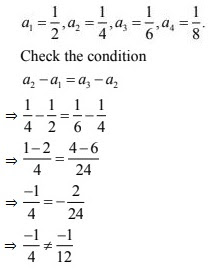Condition is not satisfied
∴ Given sequence not in A.P.

(iv) 12, 2, -8, -18, .........
In the given sequence
a1 = 12, a2 = 2, a3 = -8, a4 = -18
Check the condition
a2 - a1 = a3 - a2
⇒ 2 - 12 = -8 - 2
⇒ -10 = -10
∴ Given sequence is in A.P. with common difference d = 10

(v) 3, 3, 3, 3, ........
In the given sequence
a1 = 3, a2 = 3, a3 = 3, a4 = 3
Check the condition
a2 - a1 = a3 - a2
⇒ 3 - 3 = 3 - 3
⇒ 0 = 0
∴ Given sequence is in A.P. with common difference d = 0

(vi) P, P + 90, P + 80, P + 270, .......where P = (999)
In the given sequence
a1 = P, a2 = p + 90, a3 = p+180, a4 = P+ 270
Check the condition
a2 - a1 = a3 - a2
⇒ P + 90 - P = P + 180 - P - 90
⇒ 90 = 180 - 90
⇒ 90 = 90
∴ Given sequence is in A.P. with common difference d = 90

(vii) 1.0, 1.7, 2.4, 3.1, ......
In the given sequence
a1 = 1.0, a2 = 1.7, a3 = 2.4, a4 = 3.1
Check the condition
a2 - a1 = a8 - a2
⇒ 1.7 - 1.0 = 2.4 - 1.7
⇒ 0.7 = 0.7
∴ The given sequence is in A.P with d = 0.7

(viii) -225, -425, -625, -825,.....
In the given sequence
a1 = -225, a2 = -425, a3 = -625, a4 = -825
Check the condition
a2 - a1 = a3 - a2
⇒ -425 + 225 = -625 +425
⇒ -200 = -200
∴ The given sequence is in A.P. with d = -200

(ix) 10, 10 + 25 , 10 + 26 , 10 + 27 , .......
In the given sequence
a1 = 10, a2 = 10 + 25, a3 = 10 + 26, a4 = 10 + 27
Check the condition
a2 - a1 = a3 - a2
⇒ 10 + 25 - 10 = 10 + 26 - 10 + 25
⇒ 25 ≠ 26 - 25
∴ The given sequence is not in A.P.

11. Justify whether it is true to say that the sequence, having following nth term is an A.P.
(i) an = 2n – 1
(ii) an = 3n² + 5
(iii) an = 1 + n + n²
Solution